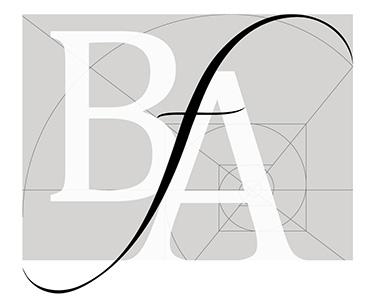# Creating Conditional forms and copying the conditions when adding a row

•bellevuefineart
Asked on February 08, 2018 at 01:39 AM

I've got this online form where people can indicate numerous files and with each file you select a size and quantity. I've built this crazy matrix to do the calculation on the first two rows. But my question is, can I copy rows in such a way that the conditionals go with them. Or is there a way to hand edit or code those conditional statements. That would be faster. I want to add rows for up to ten images, each row appears if the row above it is filled out, also using a conditional statement for show multiple ...There has to be a way without my needing to hand code this for each row•Answered on February 08, 2018 at 06:19 AM

You can achieve that with one conditional instead of 100+ with the help of calculation values.

First, assign the correct calculation values to the Size drop-down.After that, you can remove all the calculation conditionals and add just this one.You can see it in action for Image 1 here: https://form.jotform.com/80382256322957

By assigning calculation values, all the calculations are made using this one conditional.

Help Article: How to Assign Calculation Value

Help Article: How to Insert Text or Calculation into a Field Using Conditional Logic

Let us know if you need further help.

•bellevuefineart
Answered on February 08, 2018 at 11:42 AM

This is helpful, but the price is not consistent. It goes down with quantity. Is there a way in calculations to use If greater than or less than? That would solve my problem. If < 100 but not great than 150, if > 150 but less than 250 etc.

Is there a way to do that?

•david
Answered on February 08, 2018 at 12:43 PM

You could use an extra number field to determine the price.  You would use conditionally update a number field with the price based on the quantity selected:

https://www.jotform.com/help/268-How-to-Insert-Text-or-Calculation-into-a-Field-Using-Conditional-Logic

Then use that price in your calculations.  If quantity < 100 update price field to x.  In the calculation, x * quantity.